CBSE Class 10 Sample Paper for 2024 Boards - Maths Standard

Class 10
Solutions of Sample Papers for Class 10 Boards

## How many throws Sanjitha practiced on 11^th day of the camp?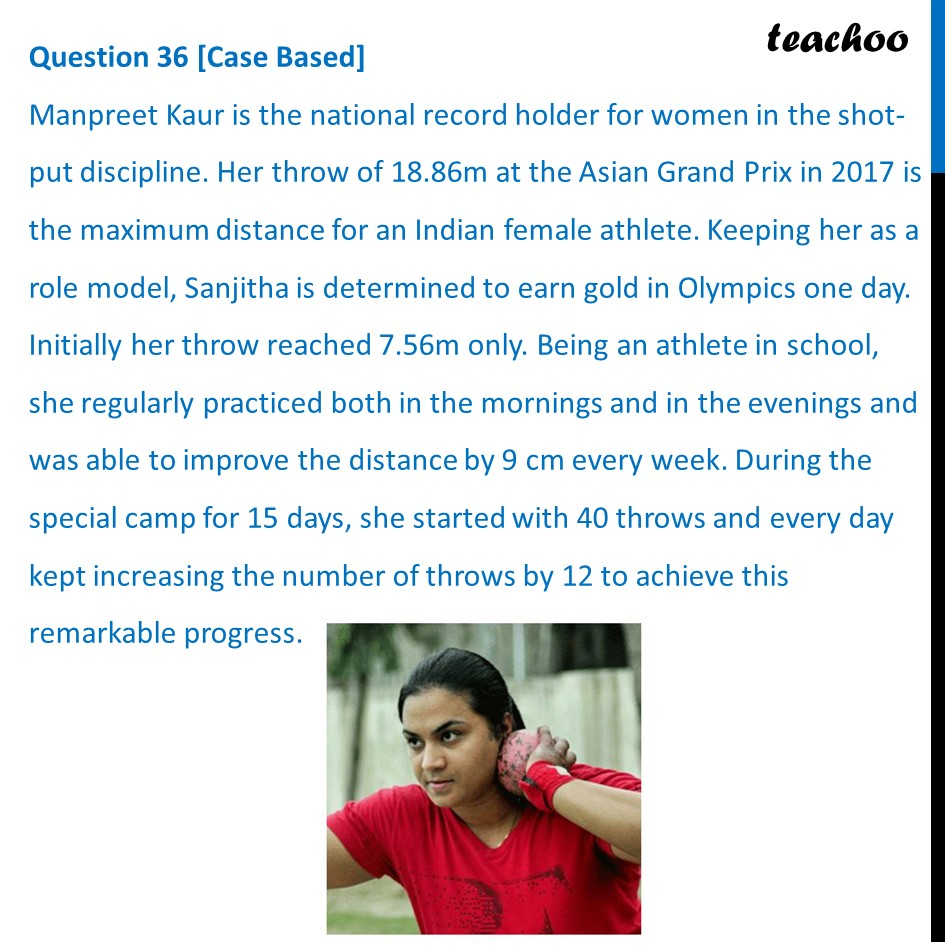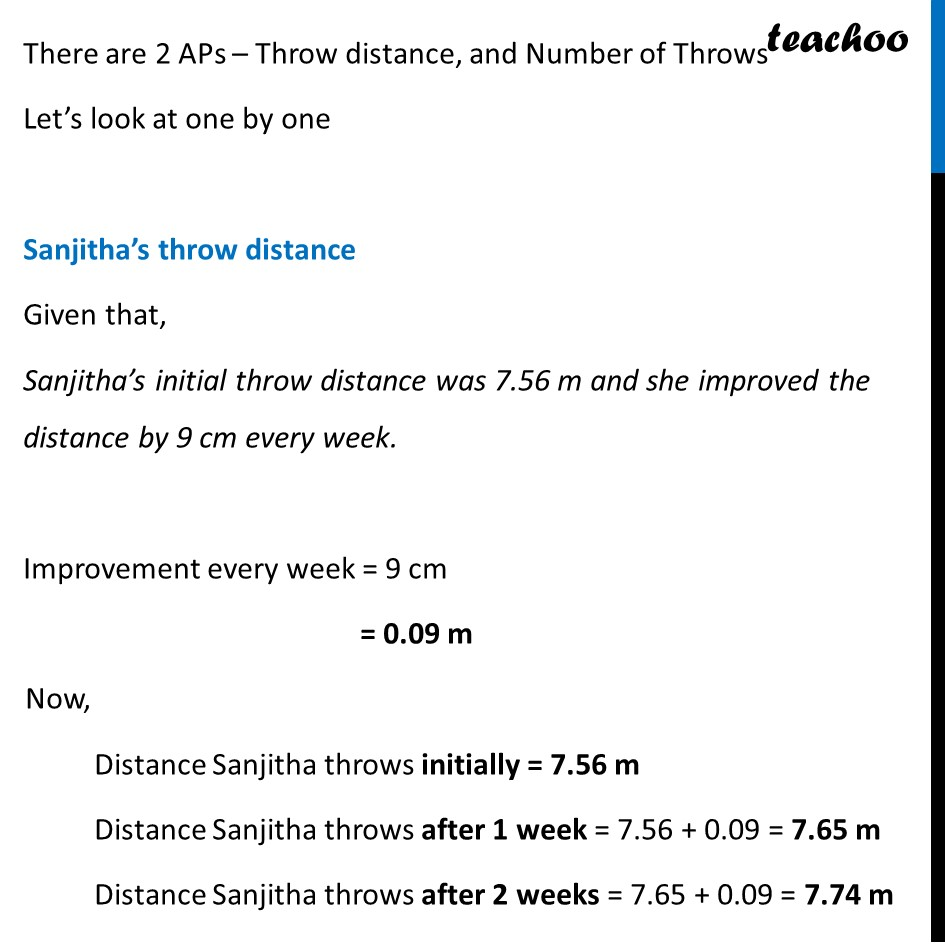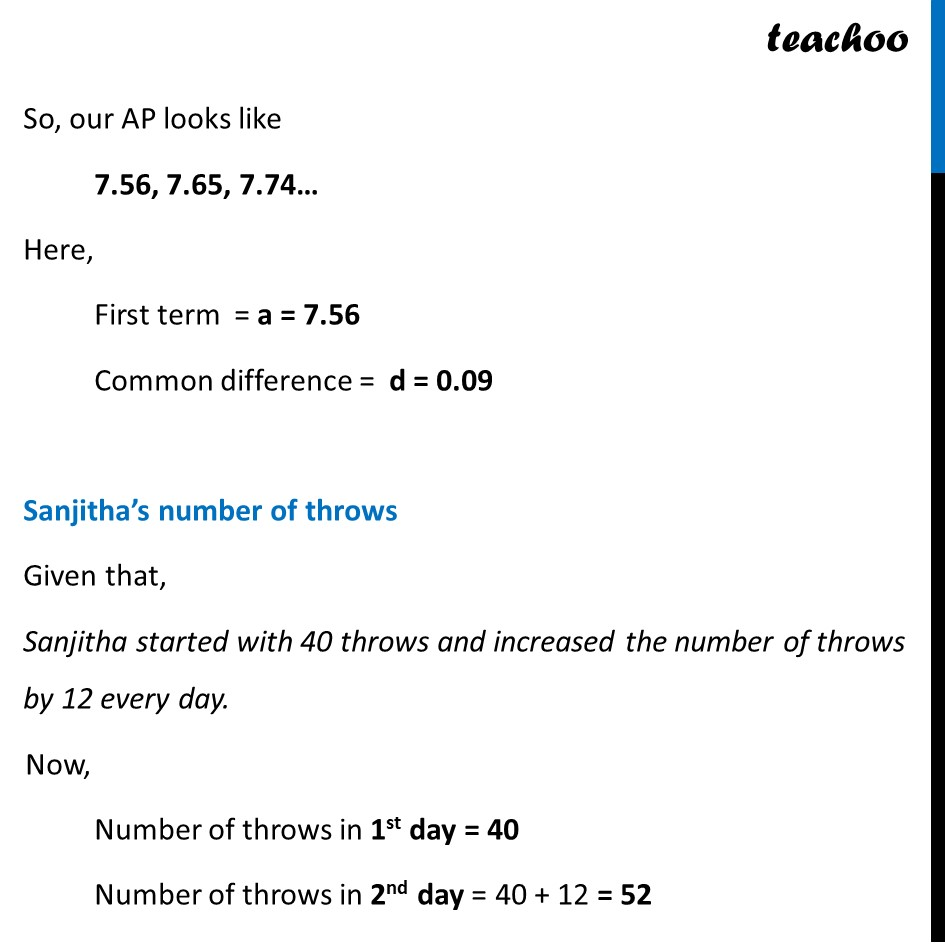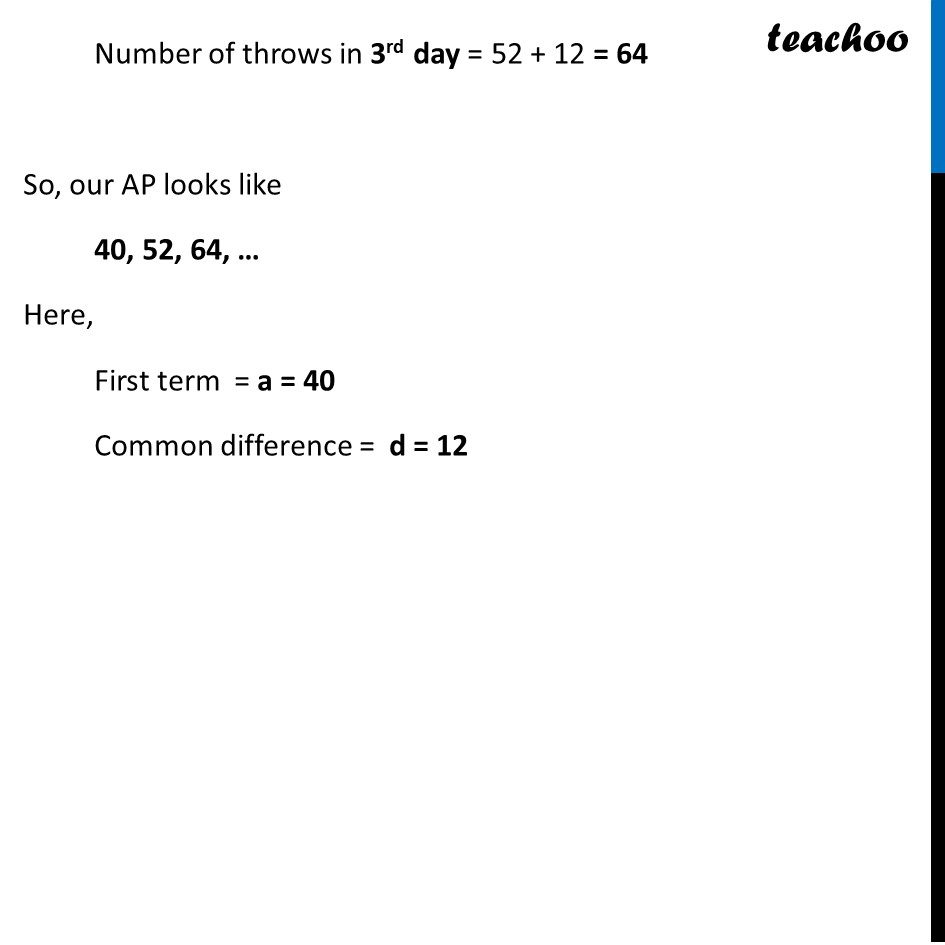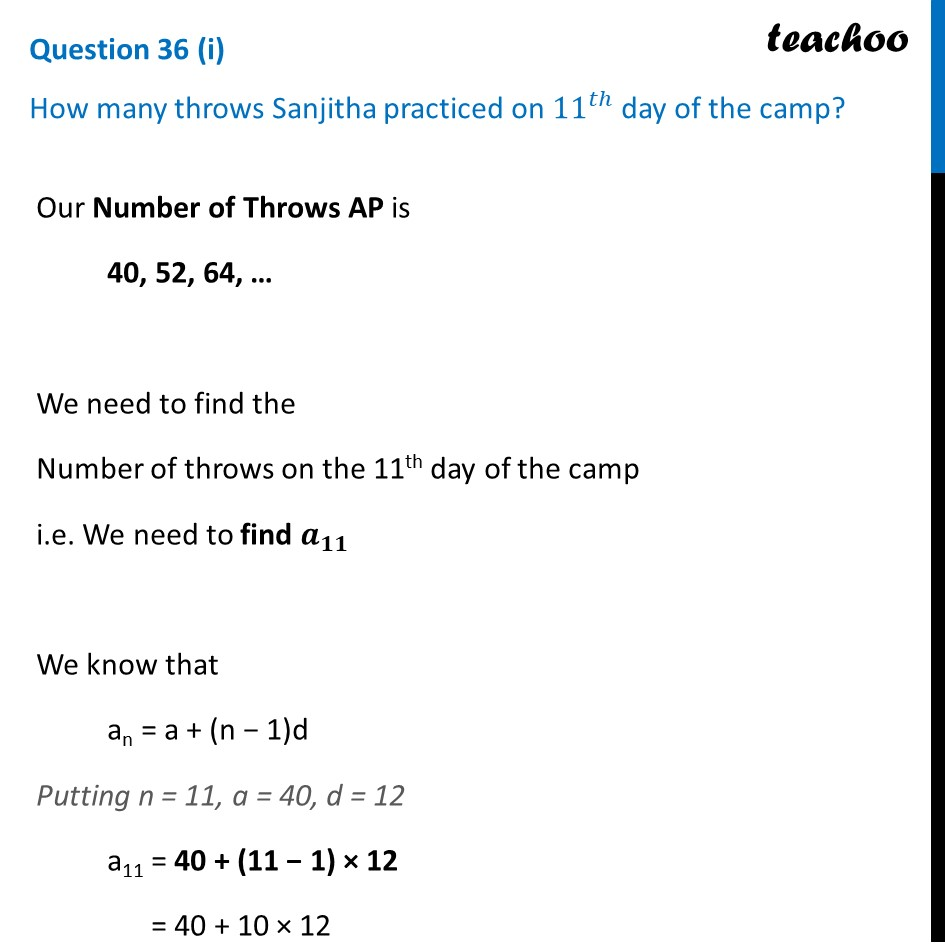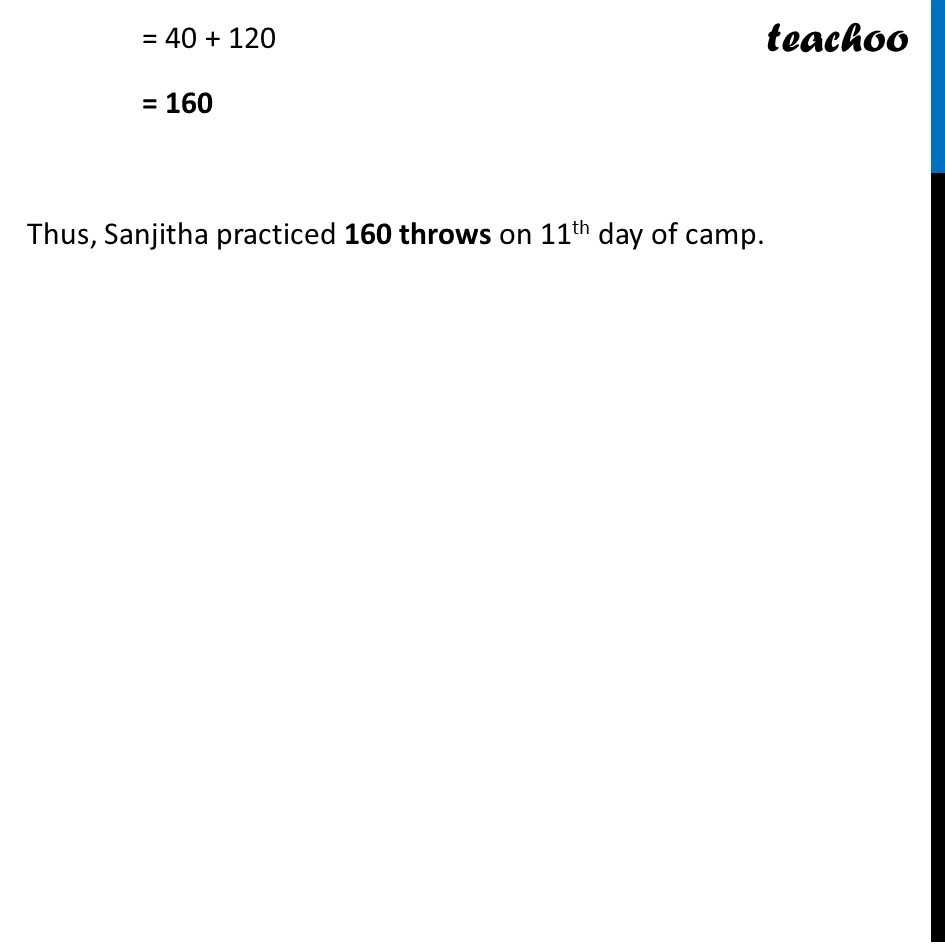Learn in your speed, with individual attention - Teachoo Maths 1-on-1 Class

### Transcript

There are 2 APs – Throw distance, and Number of Throws Let’s look at one by one Sanjitha’s throw distance Given that, Sanjitha’s initial throw distance was 7.56 m and she improved the distance by 9 cm every week. Improvement every week = 9 cm = 0.09 m Now, Distance Sanjitha throws initially = 7.56 m Distance Sanjitha throws after 1 week = 7.56 + 0.09 = 7.65 m Distance Sanjitha throws after 2 weeks = 7.65 + 0.09 = 7.74 m So, our AP looks like 7.56, 7.65, 7.74… Here, First term = a = 7.56 Common difference = d = 0.09 Sanjitha’s number of throws Given that, Sanjitha started with 40 throws and increased the number of throws by 12 every day. Now, Number of throws in 1st day = 40 Number of throws in 2nd day = 40 + 12 = 52 Number of throws in 3rd day = 52 + 12 = 64 So, our AP looks like 40, 52, 64, … Here, First term = a = 40 Common difference = d = 12 Our Number of Throws AP is 40, 52, 64, … We need to find the Number of throws on the 11th day of the camp i.e. We need to find 𝒂_𝟏𝟏 We know that an = a + (n − 1)d Putting n = 11, a = 40, d = 12 a11 = 40 + (11 − 1) × 12 = 40 + 10 × 12 = 40 + 120 = 160 Thus, Sanjitha practiced 160 throws on 11th day of camp.